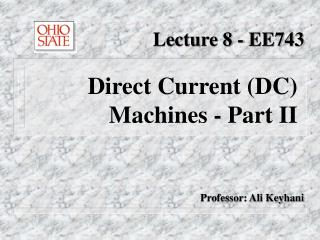Download PresentationLecture 8 - EE743

Loading in 2 Seconds...

# Lecture 8 - EE743 - PowerPoint PPT Presentation

Direct Current (DC) Machines - Part II. Lecture 8 - EE743. Professor: Ali Keyhani. DC Machines. Shunt-connected DC Machine. DC Machines. The dynamic equations (assuming r fext =0) are:. Where L ff = field self-inductance L la = armature leakage inductanceI am the owner, or an agent authorized to act on behalf of the owner, of the copyrighted work described.
Download Presentation## Lecture 8 - EE743

An Image/Link below is provided (as is) to download presentation

Download Policy: Content on the Website is provided to you AS IS for your information and personal use and may not be sold / licensed / shared on other websites without getting consent from its author.While downloading, if for some reason you are not able to download a presentation, the publisher may have deleted the file from their server.

- - - - - - - - - - - - - - - - - - - - - - - - - - E N D - - - - - - - - - - - - - - - - - - - - - - - - - -
Presentation Transcript
1. Direct Current (DC) Machines - Part II Lecture 8 - EE743 Professor: Ali Keyhani

2. DC Machines • Shunt-connected DC Machine

3. DC Machines • The dynamic equations (assuming rfext=0) are: Where Lff = field self-inductance Lla= armature leakage inductance Laf = mutual inductance between the field and rotating armature coils ea = induced voltage in the armature coils (also called counter or back emf )

4. DC Machines

5. DC Machines - Shunt DC Machine • Time-domain block diagram • The machine equations are solved for:

6. DC Machines - Shunt DC Machine • Time domain block diagram + - Va ia G2 if Vf G1 Laf X + - Vf G3 ia X if

7. DC Machines - Shunt DC Machine • State-space equations Let ; Re-writing the dynamic equations,

8. DC Machines - Permanent Magnet • The field flux in the Permanent Magnet machines is produced by a permanent magnet located on the stator. • Therefore, • Lsfif is a constant determined by the strength of the magnet, the reluctance of the iron, and the number of turns of the armature winding.

9. DC Machines - Permanent Magnet • Dynamic equations of a Permanent Magnet Machine

10. DC Machines - Permanent Magnet • Dynamic equations,

11. DC Machines - Permanent Magnet • Time domain block diagram • The equations are solved by,

12. DC Machines - Permanent Magnet • Time domain block diagram Va + - r Te ia + - ea Kv G2 G1 TL Kvr Kv

13. DC Machines - Permanent Magnet • State-space equations • re-writing the equations as function of states,

14. DC Machines - Permanent Magnet • In a matrix form,

15. DC Machines - Permanent Magnet • Transfer Function, • Let

16. DC Machines - Permanent Magnet • The, we will have • Re-arranging the equation,

17. DC Machines - Permanent Magnet • In a matrix representation,

18. DC Machines - Permanent Magnet • Solving for ia

19. DC Machines - Permanent Magnet • Let m be, • The equation is then reduced to,

20. DC Machines - Permanent Magnet

21. DC Machines - Permanent Magnet

22. DC Machines - Permanent Magnet • The characteristic equation (or force-free equation) of the system is as shown below,

23. DC Machines - Permanent Magnet • If  < 1 , the roots are a conjugate complex pair, and the natural response consists of an exponentially decaying sinusoids. • If  > 1, the roots are real and the natural response consists of two exponential terms with negative real exponents.